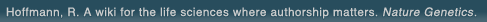# The world's first wiki where authorship really matters (Nature Genetics, 2008). Due credit and reputation for authors. Imagine a global collaborative knowledge base for original thoughts. Search thousands of articles and collaborate with scientists around the globe.

wikigene or wiki gene protein drug chemical gene disease author authorship tracking collaborative publishing evolutionary knowledge reputation system wiki2.0 global collaboration genes proteins drugs chemicals diseases compound
Hoffmann, R. A wiki for the life sciences where authorship matters. Nature Genetics (2008)# Nonexercise models for estimating VO2max with waist girth, percent fat, or BMI.

Previously published nonexercise models using either percent fat or body mass index (BMI) as body composition measures provided valid estimates of VO2max. PURPOSE: This study was conducted to investigate the use of waist girth (WG) as a body composition surrogate in the nonexercise models and to compare the accuracy of nonexercise models that include WG, %fat, or BMI. METHODS: A total of 2417 men and 384 women were measured for VO2max by indirect calorimetry ( RER > 1.1); age (yr); gender by M = 1, W = 0; self-report activity habit by the 11-point (0-10) NASA physical activity status scale (PASS); WG at the apex of the umbilicus; %fat by skinfolds; and BMI by weight (kg) divided by height squared (m). RESULTS: Three models were developed by multiple regression to estimate VO2max from age, gender, PASS, and either WG (R = 0.81, standard error of estimate (SEE) = 4.80 mL.kg.min), %fat (R = 0.82, SEE = 4.72 mL x kg(-1) x min(-1)), or BMI (R = 0.80, SEE = 4.90 mL x kg(-1) x min(-1)). Cross-validation by the PRESS technique confirmed these statistics. Accuracy of the models for predicting VO2max of subsamples was supported by constant errors (CE) < 1 mL.kg.min for subgroups of gender, age, PASS, and VO2max between 30 and 50 mL x kg(-1) x min(-1) (70% of the sample). CE were > 1 mL x kg(-1) x min(-1) for VO2max < 30 and > 50 mL x kg(-1) x min(-1). CONCLUSIONS: Waist girth is an acceptable surrogate for body composition in the nonexercise models. All models were similar in accuracy and valid for estimating VO2max of most adults, but with reduced accuracy at the extremes of fitness (VO2max < 30 and >50 mL x kg(-1) x min(-1)).

## References

1. Nonexercise models for estimating VO2max with waist girth, percent fat, or BMI. Wier, L.T., Jackson, A.S., Ayers, G.W., Arenare, B. Medicine and science in sports and exercise. (2006) [Pubmed]